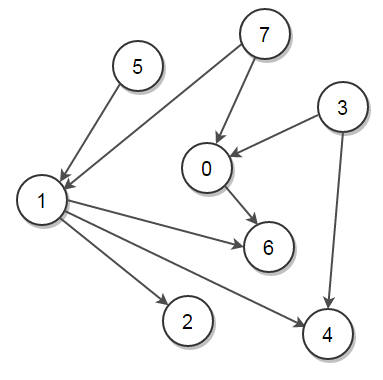# Find all Possible Topological Orderings of a DAG

Given a Directed Acyclic Graph (DAG), print all its topological orderings.

A topological ordering of a directed graph G is a linear ordering of the nodes as v1,v2,..,vn such that all edges point forward: for every edge (vi,vj), we have i < j. Moreover, the first node in a topological ordering must be one that has no edge coming into it. Analogously, the last node must be one that has no edge leaving it. A topological order is only possible when the graph has no directed cycles, i.e. if the graph is DAG.

For example, consider below graphAbove graph has many valid topological ordering of vertices like,

7, 5, 3, 1, 4, 2, 0, 6
7, 5, 1, 2, 3, 4, 0, 6
5, 7, 3, 1, 0, 2, 6, 4
3, 5, 7, 0, 1, 2, 6, 4
5, 7, 3, 0, 1, 4, 6, 2
7, 5, 1, 3, 4, 0, 6, 2
5, 7, 1, 2, 3, 0, 6, 4
3, 7, 0, 5, 1, 4, 2, 6

.. and many more

Note that for every directed edge u -> v, u comes before v in the ordering. For example, the pictorial representation of the topological order {7, 5, 3, 1, 4, 2, 0, 6} is:In the previous post, we have seen how to print topological order of a graph using Depth First Search (DFS) algorithm. We have also seen Kahn’s Topological Sort Algorithm which provides a efficient way to print topological order of a graph. In this post, we will see how to print all possible topological orderings of a DAG.

The idea remains similar to the Kahn's Topological Sort where we find vertices with no incoming edges and removing all outgoing edges from these vertices. We build all possible orderings from left to right, where the vertices with in-degree zero become candidates for the next vertex. This can be done using backtracking where the graph state is restored after processing the selected vertex.

The algorithm can be implemented as follows in C++:(2 votes, average: 5.00 out of 5)Loading...

Please use our online compiler to post code in comments. To contribute, get in touch with us.
Like us? Please spread the word and help us grow. Happy coding 🙂